### Home > INT2 > Chapter 12 > Lesson 12.2.4 > Problem12-140

12-140.
1. Graph and connect the points G(–2, 2), H(3, 2), I(6, 6), and J(1, 6) to form GHIJ. 12-140 HW eTool (Desmos). Desmos Accessibility Homework Help ✎

1. What specific type of shape is quadrilateral GHIJ? Justify your conclusion

2. Write the equations of the diagonals GI and HJ.

3. Compare the slopes of the diagonals. How do the diagonals of a rhombus appear to be related?

4. Determine the coordinates of point J' if quadrilateral GHIJ is rotated 90° clockwise (↻) about the origin.

5. Calculate the area of quadrilateral GHIJ.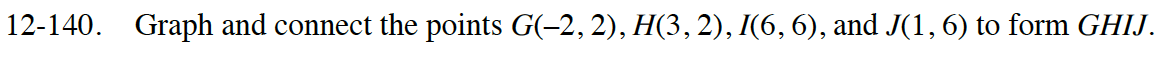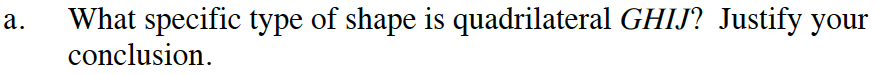Use the Pythagorean Theorem and the triangles shown to determine the lengths of GJ and HI.

What type of quadrilateral (other than a square) has sides all of the same length?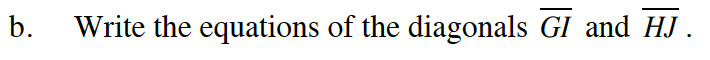Use slope triangles to find the equations for the diagonals.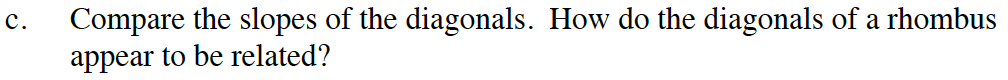If one line has a slope that is the opposite of the reciprocal of the slope of another line, the two lines are perpendicular.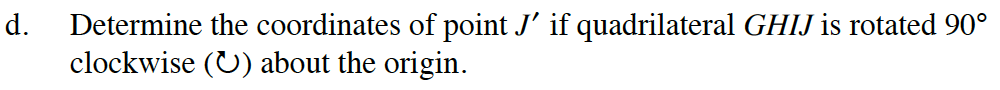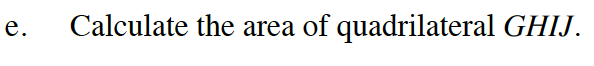Area of a parallelogram = (b)(h).

Move the points in the eTool to their given coordinates. The shape will appear when all the points are in their proper places.
Click the link at right for the full eTool version: Int2 12-140 HW eTool